# PROWAREtech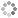Create a Bitmap Image from within the MVC controller and send it to the browser; written in C# and compatible with Linux and Windows servers.

Download any file type from a controller in ASP.NET MVC using `File`.

This example creates a bitmap (jpeg) and sends it to the browser. It requires the NuGet package SixLabors.ImageSharp v1.0.4.

The code that follows will create and then alternate between these four images: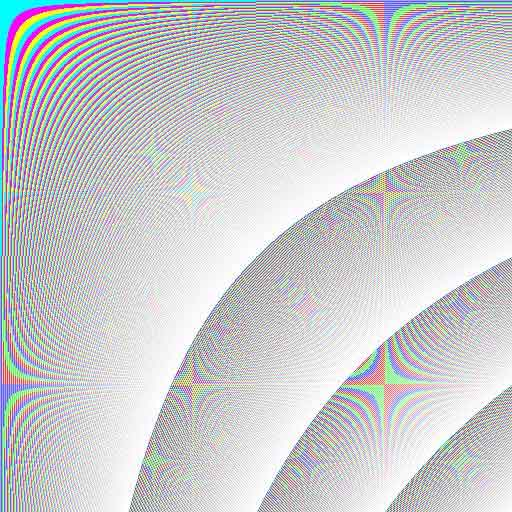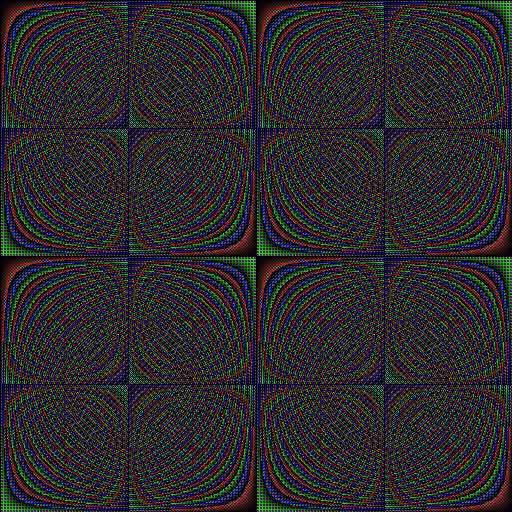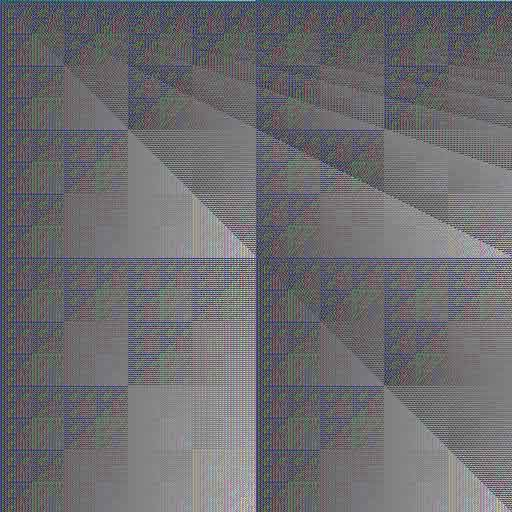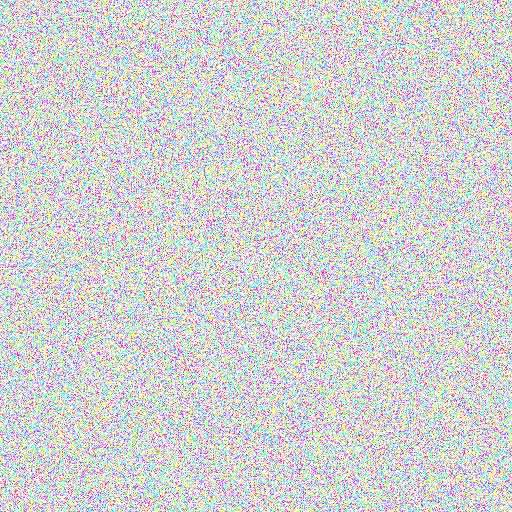The modified HomeController.cs file:

``````
// HomeController.cs
using Microsoft.AspNetCore.Mvc;
using SixLabors.ImageSharp;
using SixLabors.ImageSharp.PixelFormats;

namespace WebApplication1.Controllers
{
public class HomeController : Controller
{
static private byte mod(int x, int y) // NOTE: only returns values 0 to 255
{
if ((y & 1) == 0) // NOTE: then base of 2 - no need for division
return (byte)(x & (y - 1));
else
return (byte)(x % y);
}
static private void Image0(Image<Rgb24> bmp) // NOTE: this will create a colorful bitmap with colorful stars
{
// NOTE: loop through every pixel of the bitmap
for (int x = 0; x < bmp.Width; x++)
for (int y = 0; y < bmp.Height; y++)
{
byte p = (byte)(x * y >> 8), c = mod(p, 3), z = 255; // NOTE: "z" can be any shade between 0 and 255
bmp[x, y] = new Rgb24(c == 0 ? p : z, c == 1 ? p : z, c == 2 ? p : z); // NOTE: set the color of the pixel at (x, y)
}
}
static private void Image1(Image<Rgb24> bmp) // NOTE: this will create a bitmap with a pattern that repeats
{
// NOTE: loop through every pixel of the bitmap
for (int x = 0; x < bmp.Width; x++)
for (int y = 0; y < bmp.Height; y++)
{
byte p = mod(x * y, 256), c = mod(p, 3), z = 0; // NOTE: "z" can be any shade between 0 and 255
bmp[x, y] = new Rgb24(c == 0 ? p : z, c == 1 ? p : z, c == 2 ? p : z); // NOTE: set the color of the pixel at (x, y)
}
}
static private void Image2(Image<Rgb24> bmp) // NOTE: this will create a bitmap with a unique diagonal pattern
{
// NOTE: loop through every pixel of the bitmap
for (int x = 0; x < bmp.Width; x++)
for (int y = 0; y < bmp.Height; y++)
{
byte p = mod(x, y), c = mod(p, 3), z = 127; // NOTE: "z" can be any shade between 0 and 255
bmp[x, y] = new Rgb24(c == 0 ? p : z, c == 1 ? p : z, c == 2 ? p : z); // NOTE: set the color of the pixel at (x, y)
}
}
static private void Image3(Image<Rgb24> bmp) // NOTE: this will create a bitmap of noise
{
var rand = new Random();
// NOTE: loop through every pixel of the bitmap
for (int x = 0; x < bmp.Width; x++)
for (int y = 0; y < bmp.Height; y++)
{
byte p = mod(rand.Next(), 256), c = mod(p, 3), z = 255; // NOTE: "z" can be any shade between 0 and 255
bmp[x, y] = new Rgb24(c == 0 ? p : z, c == 1 ? p : z, c == 2 ? p : z); // NOTE: set the color of the pixel at (x, y)
}
}
public IActionResult Index()
{
int image = int.Parse(Request.Cookies["image"] ?? "0");
const int shift = 9;
const int width = 1 << shift, height = 1 << shift;
using (var bmp = new Image<Rgb24>(width, height)) // NOTE: create 24-bit bitmap
{
switch (mod(image, 4))
{
case 0:
Image0(bmp);
break;
case 1:
Image1(bmp);
break;
case 2:
Image2(bmp);
break;
case 3:
Image3(bmp);
break;
}
byte[] data;
using (var ms = new MemoryStream())
{
bmp.SaveAsJpeg(ms); // NOTE: save the bitmap as a JPEG image to the stream
data = ms.ToArray();

}
// NOTE: alternatively, send the data to the browser as a download with the file name "attachment.jpg" by uncommenting the following line
// return File(data, System.Net.Mime.MediaTypeNames.Image.Jpeg, "attachment.jpg");

return File(data, System.Net.Mime.MediaTypeNames.Image.Jpeg); // NOTE: send the data to the browser as a file of mime type "image/jpeg"
}
}
}
}
``````This site uses cookies. Cookies are simple text files stored on the user's computer. They are used for adding features and security to this site. Read the privacy policy.